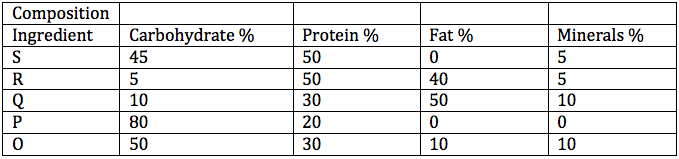Data Interpretation and Logical Reasoning Questions for CAT

2
10211

Data Interpretation and logical reasoning is claimed to be the most difficult by many CAT aspirants. But with adequate practice if you can change your score drastically by getting one more set right in either DI or LR CAT questions. We give some important Logical reasoning set based from previous papers.

Data Interpretation and Logical Reasoning Questions for CAT:

Instructions:

A health-drink company’s R&D department is trying to make various diet formulations, which can be used for certain specific purposes. It is considering a choice of 5 alternative ingredients (O, P, Q, R, and S), which can be used in different proportions in the formulations.

The table below gives the composition of these ingredients. The cost per unit of each of these ingredients is O: 150, P: 50, Q: 200, R: 500, S: 100.Question 1:

For a recuperating patient, the doctor recommended a diet containing 10% minerals and at least 30% protein. In how many different ways can we prepare this diet by mixing at least two ingredients?

a) One
b) Two
c) Three
d) Four
e)None

Question 2:

Which among the following is the formulation having the lowest cost per unit for a diet having 10% fat and at least 30% protein? The diet has to be formed by mixing two ingredients.

a) P and Q
b) P and S
c) P and R
d) Q and S
e)R and S

Question 3:

In what proportion P, Q and S should be mixed to make a diet having at least 60% carbohydrate at the lowest per unit cost?

a) 2 : 1 : 3
b) 4 : 1 : 2
c) 2 : 1 : 4
d) 3 : 1: 2
e)4 : 1 : 1

Download the CAT Verbal Ability PDFs/Ebooks and all Quantitative aptitude questions PDF.

Question 4:

In what proportion P, Q and S should be mixed to make a diet having at least 60% carbohydrate at the lowest per unit cost?

a) 2 : 1 : 3
b) 4 : 1 : 2
c) 2 : 1 : 4
d) 3 : 1: 2
e)4 : 1 : 1

Question 5:

The company is planning to launch a balanced diet required for growth needs of adolescent children. This diet must contain at least 30% each of carbohydrate and protein, no more than 25% fat and at least 5% minerals. Which one of the following combinations of equally mixed ingredients is feasible?

a) O and P
b) R and S
c) P and S
d) Q and R
e)O and S

Solutions (1 to 5)

We want a diet with 10% minerals and at least 30% protien. The mixture of O and Q is suitable because both contain 10% minerals.In required proportion we can get 10% minerals and at least 30% proteins.
Solution P and R contains minerals which are less than 10% and maximum mineral %age in any component is 10. So if we include P or R in any mixture,we can’t get 10% mineral %age.
So only 1 solution of O and Q is possible.

Option A: P and Q in 4:1 ratio gives fat 10% but the protein is 22%
Option B: P and S do not contain fat. Hence we wont analyse the option.
Option C: P and R in 3:1 ratio gives fat 10% but the protein is 27.5%
Option D: Q and S in 1:4 gives fat and protein as 10% and 46 %.
Cost of the mixture= [(1*200)+(4*100)]/5=120
Option E: R and S in 1:3 gives 10% fat and 50%protein.
Cost of the mixture= [(1*500)+(3*100)]/4=200

P,Q,S contain 80%,10% and 45% carbohydrates respectively.
Option A,C and D does not contain 60% carbohydrates.
Option E: Carbohydrate %=[(4*80)+(1*10)+(1*45)]/6= 62.5%
Cost per unit=[(4*50)+(200)+100]/6=83.3
Option B: Carbohydrate %=[(4*80)+(1*20)+(2*45)]/7= 61.4%
Cost per unit=[(4*50)+(200)+(2*100)]/7=600/7=85.7